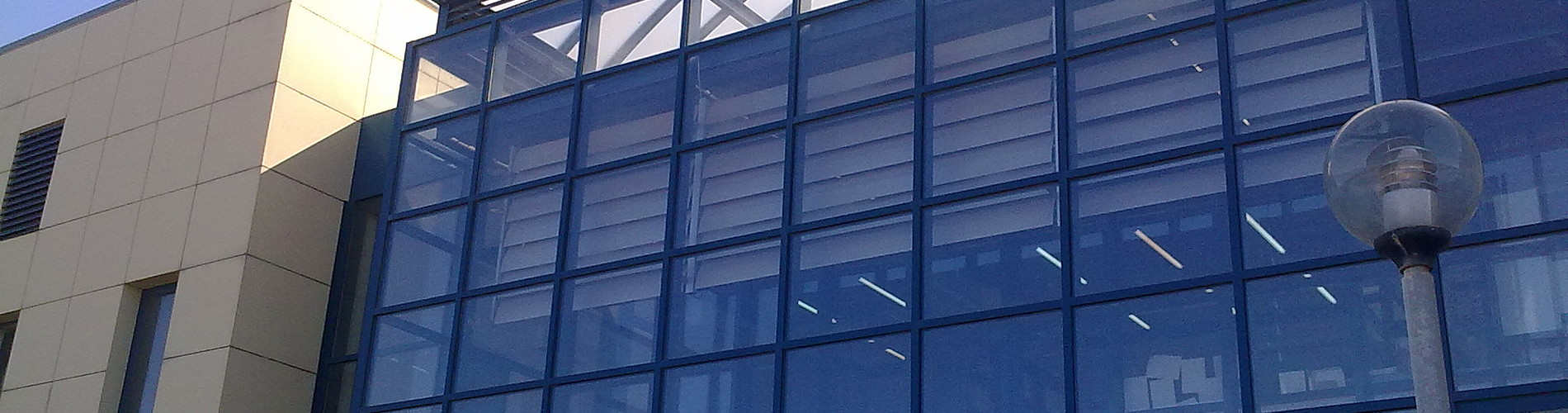School of Chemical and Environmental Engineering

Now offering two distinct diplomas: Chemical Engineering and Environmental Engineering

# Differential and Integral Calculus I

1. COURSE INFORMATION:

 School Environmental Engineering Course Level Undergraduate Course ID MATH 101 Semester 1st Course Category Required Course Modules Instruction Hours per Week ECTS Lectures and Tutorial Exercises 4 Th=3, E=1, L=0 5 Course Type General Background Prerequisites Instruction/Exam Language Greek The course is offered to Erasmus students No Course URL https//www.eclass.tuc.gr/courses/MHPER314/ (in Greek)

2. LEARNING OUTCOMES

 Learning Outcomes This course aims to provides the essential mathematical techniques that an engineer needs in the theoretical part of his training as well as  what he/she  shall need in the computational part of his work. On completion of this course students will be expected to apply the mathematical induction method calculate limits of sequences and examine the convergence of series compute derivatives and antiderivatives of functions  find the extreme values of functions and solve optimization problems  sketch the graph of functions to evaluate  integrals using the basic techniques of integration evaluate areas, volumes of solids with known cross sections, moments and center of mass, the work done by a variable force along the x-axis, the fluid force against a  vertical flat plate. approximate functions by Taylor polynomial and calculate the errors bound represent   certain functions as series and  evaluate the   values at a point up a desired error. General Competencies/Skills Review, analyse and synthesise data and information Work autonomously Work in teams Work in an international frame Advance free, creative  and causative thinking

3. COURSE SYLLABUS

 Real variables. Principle of Mathematical induction. Sequences, limits of sequences, recursively defined sequences. Series (Convergence criteria) Functions of one real variable. Limits and continuity of functions. Derivatives. Geometric interpretation of the derivative. Differentials. Applications of the derivative. Definite integrals. Basic theorems of integral calculus. Applications of integration: Αreas between two curves,  volumes and surfaces of solids by revolution, arc length, moments and centroids, hydrostatic pressure.  Exponential Functions. Inverse functions. Hyperbolic functions. Indefinite integral. Integration techniques. Techniques of Integration: Integration by Parts,Trigonometric Integrals, Trigonometric Substitutions,Integration of Rational Functions by Partial Fractions, Improper Integrals. Power series and Taylor series. Applications of series.

4. INSTRUCTION and LEARNING METHODS - ASSESSMENT

 Lecture Method Direct (face to face) Use of Information and Communication Technology E-class support Instruction Organisation Activity Workload per Semester (hours) - Lectures 39 - Lab assignments 13 - Autonomous study 73 Course Total 125 Assessment Method Written final examination (100 %).